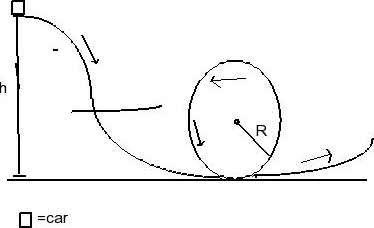# Problem: A roller coaster car may be approximated by a block of mass m. The car, which starts from rest, is released at a height above the ground and slides along a frictionless track. The car encounters a loop of radius R, as shown. Assume that the initial height is great enough so that the car never loses contact with the track.Ignore stray line on trackFind an expression for the kinetic energy of the car at the top of the loop. Express the kinetic energy in terms of m, g, h, and R.

###### FREE Expert Solution

Law of conservation of energy:

$\overline{){{\mathbf{K}}}_{{\mathbf{i}}}{\mathbf{+}}{{\mathbf{U}}}_{{\mathbf{i}}}{\mathbf{+}}{{\mathbf{W}}}_{{\mathbf{nc}}}{\mathbf{=}}{{\mathbf{K}}}_{{\mathbf{f}}}{\mathbf{+}}{{\mathbf{U}}}_{{\mathbf{f}}}}$

Wnc is the work done by non-conservative forces.

Kinetic energy:

$\overline{){\mathbf{K}}{\mathbf{=}}\frac{\mathbf{1}}{\mathbf{2}}{\mathbf{m}}{{\mathbf{v}}}^{{\mathbf{2}}}}$

Potential energy:

$\overline{){\mathbf{U}}{\mathbf{=}}{\mathbf{m}}{\mathbf{g}}{\mathbf{h}}}$

Work done by nonconservative forces is zero.###### Problem Details

A roller coaster car may be approximated by a block of mass m. The car, which starts from rest, is released at a height above the ground and slides along a frictionless track. The car encounters a loop of radius R, as shown. Assume that the initial height is great enough so that the car never loses contact with the track.

Ignore stray line on trackFind an expression for the kinetic energy of the car at the top of the loop. Express the kinetic energy in terms of m, g, h, and R.